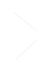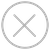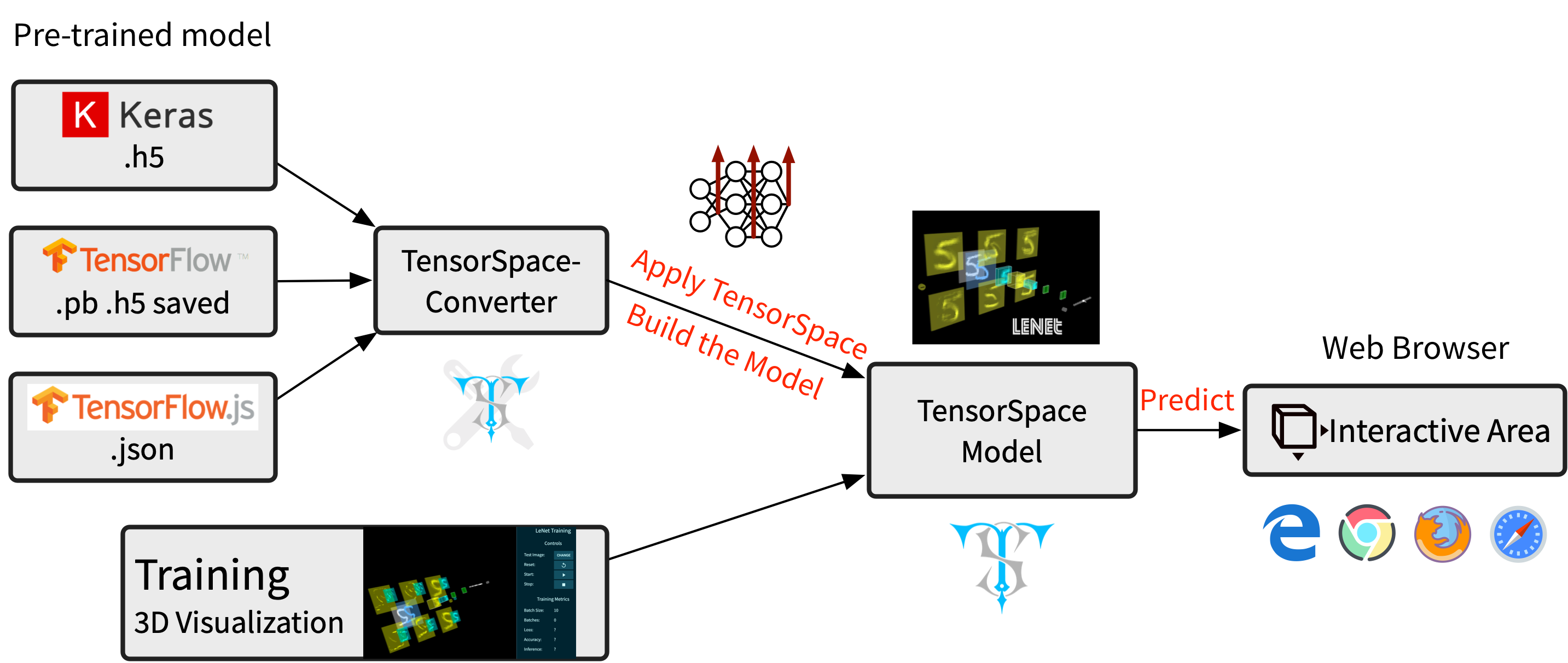TensorSpace.js
Getting StartBasic ConceptsTensorSpace ConverterModel PreprocessingModelsLayersMerge FunctionGetting Start Introduction
TensorSpace is a neural network 3D visualization framework built by TensorFlow.js, Three.js and Tween.js. TensorSpace provides Layer APIs to build deep learning layers, load pre-trained models, and generate a 3D visualization in the browser. By applying TensorSpace API, it is more intuitive to visualize and understand any pre-trained models built by TensorFlow, Keras, TensorFlow.js, etc.
Next, there are some topics we can checkout:Fig. 1 - TensorSpace Workflow